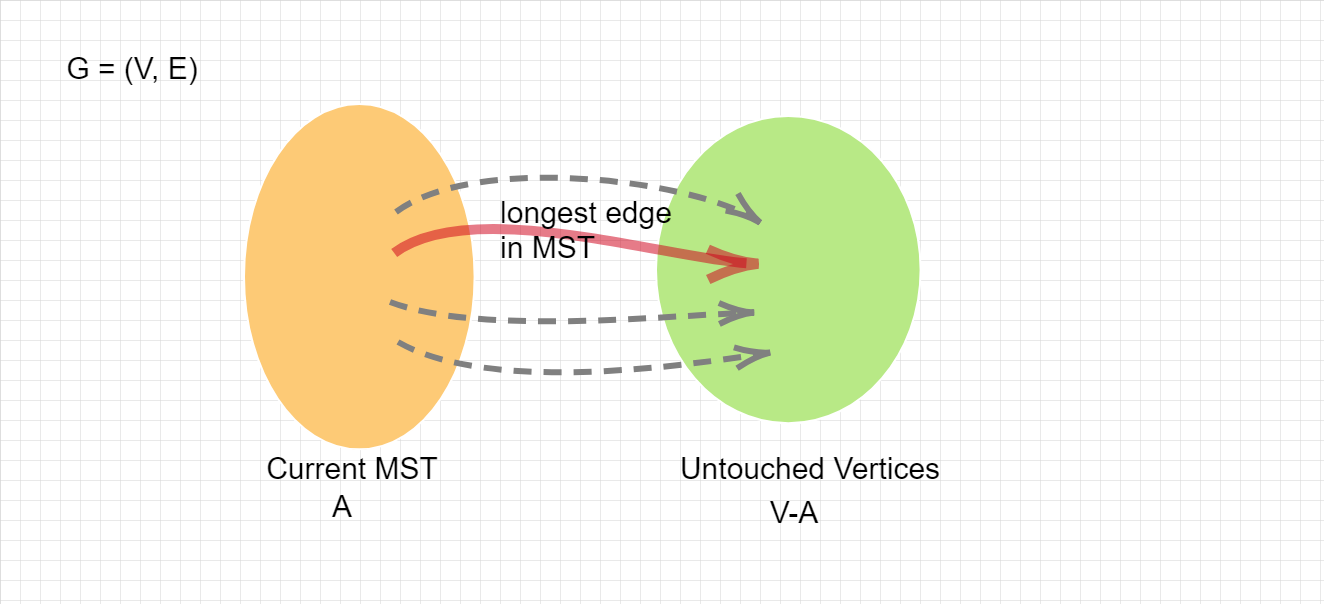# Sixian Li

## Minimum spanning tree

Algorithm

My attempt to reconstruct a detailed proof for a remark on Luc Devroye’s note of MST.

## Max edge in a spanning tree

Let $T$ be a spanning tree of $G(V,E)$ and $L(T) = max_{(u,v)\in T}\ w[u, v]$, the longest edge in a spanning tree.

$\text{Claim: The minimum spanning tree also minimizes L(T), that is, }$

$L(MST) = min_{all\ T} L(T)$

$\text{Proof:}$$\geq$

MST$\in$T, so L(MST) cannot be less than the minimum L(T) of all T’s.

$\leq$

In the figure above, we assume the red arrow is the longest edge in MST. We construct the MST by adding the shortest “bridge” each time we cross two sets, $A$ and $V-A$. Thus, all other grey edges are longer than or equal to the red edge. These grey edges are in some other spanning trees.

For convenience, take one grey edge and call it $(x,y)$ because it’s from some vertex $x\in A$ to $y \in V-A$. If it’s the max edge of its spanning tree, $T_x$. $L(T_x) \geq L(MST)$. If it’s not, there exists a max edge $(x',y')$ in $T_x$ such that $(x',y') \gt (x, y) \geq L(MST)$. So $L(T_x) \geq L(MST)$.

Q.E.D